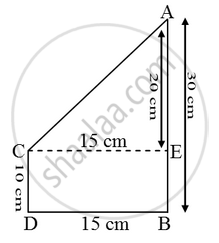Share

# Two towers of heights 10 m and 30 m stand on a plane ground. If the distance between their feet is 15 m, find the distance between their tops - CBSE Class 10 - Mathematics

#### Question

Two towers of heights 10 m and 30 m stand on a plane ground. If the distance between their feet is 15 m, find the distance between their tops

#### SolutionBy Phythagoras theorem, we have

AC^2 = CE^2 + AE^2

⇒ AC^2 = 15^2 + 20^2 = 225 + 400 = 625

⇒ AC = \sqrt { 625 } = 25 m.

Is there an error in this question or solution?

#### Video TutorialsVIEW ALL 

Solution Two towers of heights 10 m and 30 m stand on a plane ground. If the distance between their feet is 15 m, find the distance between their tops Concept: Pythagoras Theorem.
S